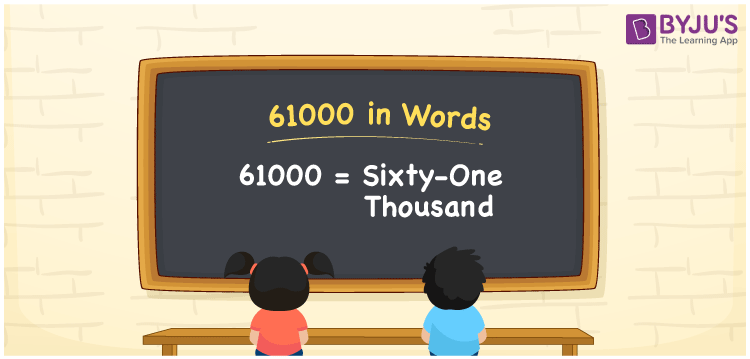# 61000 in words

61000 in words is written as Sixty One Thousand. In 61000, 6 has a place value of ten thousand and 1 has the place value of thousand. The article on Place Value gives more information. The number 61000 is used in expressions that relate to money, distance, length, population and many more. For example, “I needed to draw Sixty One Thousand Rupees from the bank”.

 61000 in words Sixty One Thousand Sixty One Thousand in Numbers 61000

## 61000 in English Words## How to Write 61000 in Words?

We can convert 61000 to words using a place value chart. The number 61000 has 5 digits, so let’s make a chart that shows the place value up to 5 digits.

 Ten thousand Thousands Hundreds Tens Ones 6 1 0 0 0

Thus, we can write the expanded form as:

6 × Ten thousand + 1 × Thousand + 0 × Hundred + 0 × Ten + 0 × One

= 6 × 10000 + 1 × 1000 + 0 × 100 + 0 × 10 + 0 × 1

= 61000

= Sixty One Thousand.

61000 is the natural number that is succeeded by 60999 and preceded by 61001.

61000 in words – Sixty One Thousand.

Is 61000 an odd number? – No.

Is 61000 an even number? – Yes.

Is 61000 a perfect square number? – No.

Is 61000 a perfect cube number? – No.

Is 61000 a prime number? – No.

Is 61000 a composite number? – Yes.

## Solved Example

1. Write the number 61000 in expanded form

Solution: 6 x 10000 + 1 x 1000 + 0 x 100 + 0 x 10 + 0 x 1

Or Just 6 x 10000 + 1 x 1000

We can write 61000 = 60000 + 1000 + 0 + 0 + 0

= 6 x 10000 + 1 x 1000 + 0 x 100 + 0 x1 0 + 0 x 1

## Frequently Asked Questions on 61000 in words

Q1

### How to write 61000 in words?

61000 in words is written as Sixty One Thousand.
Q2

### State True or False. 61000 is divisible by 3?

False. 61000 is not divisible by 3.
Q3

### Is 61000 divisible by 10?

Yes. 61000 is divisible by 10.